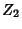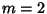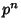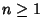## Cyclic Group

A cyclic groupof Orderis a Group defined by the element(the Generator) and itsPowers up towhereis the Identity Element. Cyclic groups are both Abelian and Simple. There exists a unique cyclic group of every order, so cyclic groups of the same order are always isomorphic (Shanks 1993, p. 74), and all Groups of Prime Order are cyclic.

Examples of cyclic groups include,,, and the Modulo Multiplication Groupssuch that, 4,, or, foran Odd Prime and(Shanks 1993, p. 92). By computing the Characteristic Factors, any Abelian Group can be expressed as a Direct Product of cyclic Subgroups, for example, Finite Group Z2Z4 or Finite Group Z2Z2Z2.

See also Abelian Group, Characteristic Factor, Finite Group Z2, Finite Group Z3, Finite Group Z4, Finite Group Z5, Finite Group Z6, Modulo Multiplication Group, Simple Group

References

Lomont, J. S. Cyclic Groups.'' §3.10.A in Applications of Finite Groups. New York: Dover, p. 78, 1987.

Shanks, D. Solved and Unsolved Problems in Number Theory, 4th ed. New York: Chelsea, 1993.